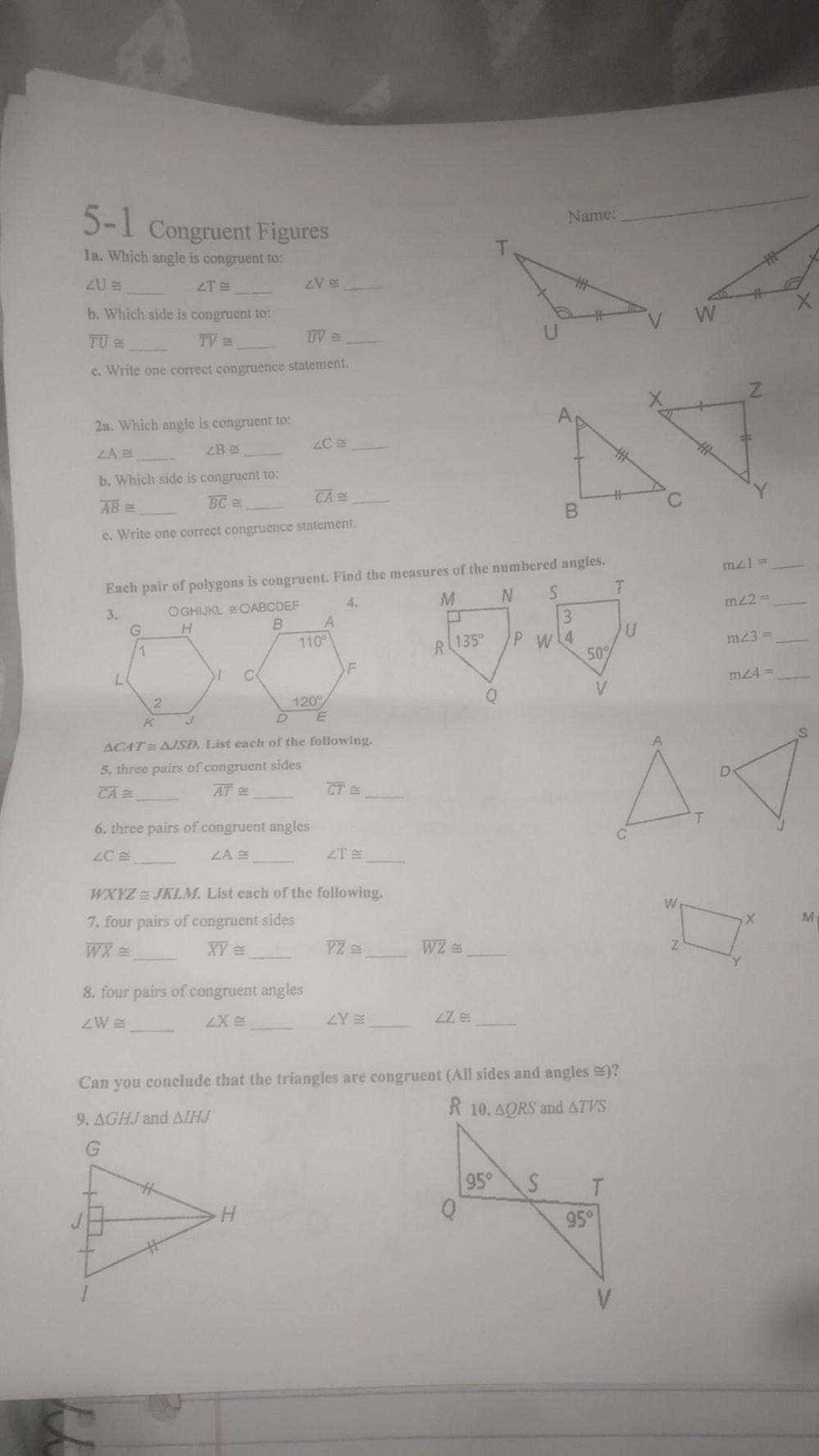Question:

# 5-1 congruent Figures Name: ZVE 1a. Which angle is congruent to: LUE LT b. Which side is congruent to TU TV w UV e c. Write one5-1 congruent Figures Name: ZVE 1a. Which angle is congruent to: LUE LT b. Which side is congruent to TU TV w UV e c. Write one correct congruence statement. Z 20. 2a. Which angle is congruent to: LAS BE b. Which side is congruent to: AB BC CA B c. Write one correct congruence statement. m2l = T m22 = Each pair of polygons is congruent. Find the measures of the numbered angles. 3. OGHIJKL & OABCDEF 4. M N S G H B A 3 1 110 IP WL4 C 50% de m23 = R(135 2 m24 = V S 2 120° K D E ACATE AISD. List each of the following. 5. three pairs of congruent sides AT = D CA CT T 6. three pairs of congruent angles LC LAS LT w WXYZ = JKLM. List each of the following. 7. four pairs of congruent sides WX XY YZ X M WZ Z 8. four pairs of congruent angles ZW LX = LY LZ Can you conclude that the triangles are congruent (All sides and angles )? 9. AGHJ and ATHI R 10. AQRS and ATVS G 95°S T 95 H